Back to Adaptive Vision Studio website

You are here: Start » Filter Reference » Image Pixel Statistics » ImageAverage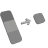ImageAverage

Computes the average of the image pixel values.

Name Type DescriptioninImage Image Input imageinRoi Region* Range of pixels to be processed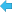outAverageColor Pixel Average of each channeloutAverageValue Real Average of the entire image

Description

The function calculates average of pixel values in the inImage.

Average values of pixels calculated for each channel are returned in outAverageColor, while calculated for the region of interest - in outAverageValue. If region of interest isn't specified, average is calculated for the entire image.

Hints

• Connect inImage with the output of your image acquisition filter.

Examples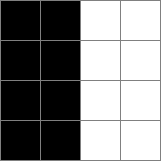In this single-channel image, average will be 127.5 for the channel and for the entire image (if the region of interested isn't specified).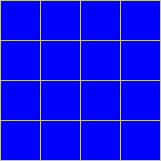In this three-channel RGB image, average will be 0 for R and G channels and 255 for the B channel. If we don't specify the region of interest, average of the image will be 85.0 (because arithmetic mean of 0, 0, and 255 is 85.0).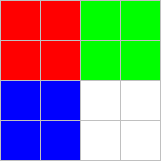In this three-channel RGB image, average will be 63.75 for R and B channels and 127.5 for the G channel. If we don't specify the region of interest, average of the image will be 85.0 (because arithmetic mean of 63.75, 63.75 and 127.5 is 85.0).

Minimal image size requirement

The input image shouldn't be empty.

Minimal region of interest size requirement

The input region of interest shouldn't be empty.

Hardware Acceleration

This operation supports automatic parallelization for multicore and multiprocessor systems.

Errors

This filter can throw an exception to report error. Read how to deal with errors in Error Handling.

List of possible exceptions:

Error type Description
DomainError Empty image on input in ImageAverage.
DomainError Empty region of interest on input in ImageAverage.

Complexity Level

This filter is available on Basic Complexity Level.